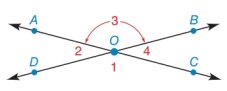Chapter 1.7, Problem 24E### Elementary Geometry for College St...

6th Edition
Daniel C. Alexander + 1 other
ISBN: 9781285195698

#### Solutions

Chapter
Section### Elementary Geometry for College St...

6th Edition
Daniel C. Alexander + 1 other
ISBN: 9781285195698
Textbook Problem
1 views

# In Exercises 19 to 26, use the drawing in which A C ↔ intersects D B ↔ at point O.If m ∠ 2 = x + 15 and m ∠ 3 = 2 x , find x and m ∠ 2 .To determine

To find:

The value of x and m2.

Explanation

Given:

The given angles are m2=x+15 and m3=2x.

The given figure is,

Definition:

The sum of measures of angles formed on straight line is supplementary angle.

Calculation:

From the given figure it can be observed that 2 and 3 are supplementary angles.

m2+m3=180°

Substitute x+15 for m2 and 2x for m3 in above equation.

### Still sussing out bartleby?

Check out a sample textbook solution.

See a sample solution

#### The Solution to Your Study Problems

Bartleby provides explanations to thousands of textbook problems written by our experts, many with advanced degrees!

Get Started

#### Convert 3,400 feet to miles.

Trigonometry (MindTap Course List)

#### If , then f−1(x) = x3 +3 (x + 3)3 x3 − 3

Study Guide for Stewart's Single Variable Calculus: Early Transcendentals, 8th

#### What can a researcher do if the treatment administered during the first B?

Research Methods for the Behavioral Sciences (MindTap Course List)